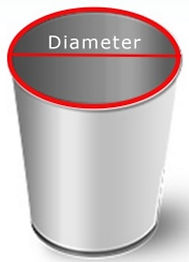## WHAT SIZE TRASH BAG DO I NEED?

###### Second, find the height...

1. Measure height of your trash can.

2. Add four inches for overhang plus half the width (diameter) to cover the bottom of your can.

Example: 55 Gallon drum

Height: 34.5"

Width/Diameter: 23"

Bag height needed: 34.5 (can height) + 4 (overhang) + 11.5 (half can width) = 50"

For square/rectangle trash cans:

1. Take the perimeter (sum of all the sides) of your trash can and divide by two. This will be the width of your trash bag.

Example: Perimeter of can = 32"

Width of trash bag = 32 (perimeter) divided by two = 16"For round trash cans:

1. Take the diameter (width of your trash can) and multiply by 3.14 (pi).

2. Take this number and divide by 2. This will be the width of your trash bag.

Example: Diameter of can = 16"

Width of trash bag = 16 x 3.14 = 50; 50 divided by 2 = 25"